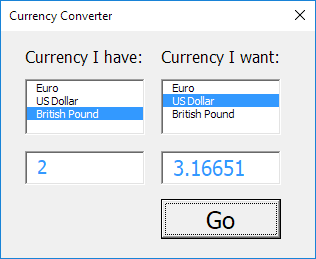# Currency Converter

Below we will look at a program in Excel VBA which creates a Userform that converts any amount from one currency into another.

The Userform we are going to create looks as follows:To create this Userform, execute the following steps.

1. Open the Visual Basic Editor. If the Project Explorer is not visible, click View, Project Explorer.

2. Click Insert, Userform. If the Toolbox does not appear automatically, click View, Toolbox. Your screen should be set up as below.3. Add the labels, list boxes (first at the left, the second at the right), text boxes (first at the left, the second at the right) and command button. Once this has been completed, the result should be consistent with the picture of the Userform shown earlier. For example, create a list box control by clicking on ListBox from the Toolbox. Next, you can drag a list box on the Userform.

4. You can change the names and the captions of the controls. Names are used in the Excel VBA code. Captions are those that appear on your screen. It is good practice to change the names of the controls, but it is not necessary here because we only have a few controls in this example. To change the caption of the Userform, command button and labels, click View, Properties Window and click on each control.

5. To show the Userform, place a command button on your worksheet and add the following code line:

Private Sub CommandButton1_Click()

UserForm1.Show

End Sub

We are now going to create the Sub UserForm_Initialize. When you use the Show method for the Userform, this sub will automatically be executed.

6. Open the Visual Basic Editor.

7. In the Project Explorer, right click on UserForm1 and then click View Code.

8. Choose Userform from the left drop-down list. Choose Initialize from the right drop-down list.

9. Add the following code lines:

Private Sub UserForm_Initialize()

With ListBox1
End With

With ListBox2
End With

ListBox1.ListIndex = 1
ListBox2.ListIndex = 0

TextBox1.Value = 1
TextBox2.Value = 0.722152

End Sub

Explanation: first, the two list boxes are filled. Next, The US Dollar currency is set as default in the first list box and the EURO currency is set as default in the second list box. Finally, the value 1 is entered in the first text box and the value 0.722152 is entered in the second text box.

We have now created the first part of the Userform. Although it looks neat already, nothing will happen yet when we click on the Go button.

10. In the Project Explorer, double click on UserForm1.

11. Double click on the Go button.

12. Add the following code lines:

Private Sub CommandButton1_Click()

Dim rates(0 To 2, 0 To 2) As Double, i As Integer, j As Integer

rates(0, 0) = 1
rates(0, 1) = 1.38475
rates(0, 2) = 0.87452

rates(1, 0) = 0.722152
rates(1, 1) = 1
rates(1, 2) = 0.63161

rates(2, 0) = 1.143484
rates(2, 1) = 1.583255
rates(2, 2) = 1

For i = 0 To 2
For j = 0 To 2
If ListBox1.ListIndex = i And ListBox2.ListIndex = j Then TextBox2.Value = TextBox1.Value * rates(i, j)
Next j
Next i

End Sub

Explanation: first, we declare an array. The array has two dimensions. It consists of 3 rows and 3 columns. Next, we initialize each element of the array. For example, rates(1,0) is the currency rate from US Dollar to EURO. Next, we start a double loop. Depending on the selected currencies in the list boxes, Excel VBA converts the amount entered in the first text box and displays the result in the second text box.

Example: if i = 2 and j = 1 and we enter the value 2 in the first text box, Excel VBA places the value 2 * rates(2,1) = 2 * 1.583255 = 3.16651 in the second text box.

13. Test the Userform.

Result:Go to Next Chapter: Range